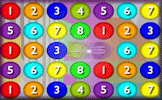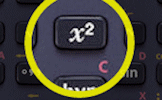# Number Systems

$$\mathbb N$$: The set of natural or counting numbers 0, 1, 2, 3, etc. Some say this set does not include zero.

$$\mathbb Z$$: The set of integers. This set is the same as the set of natural numbers but includes the negative whole numbers.

$$\mathbb Q$$: The set of rational numbers. These are the numbers that can be expressed as a proper fraction or one integer divided by another.

$$\mathbb R$$: The set of real numbers. That is probably all the numbers you know unless you have studied the topic of Complex Numbers.

$$\mathbb R$$
$$\mathbb Q$$
$$\mathbb Z$$
$$\mathbb N$$
$$\text{one million}$$
$$\frac34$$
$$\cos 40^o$$
$$\pi$$
$$\sin 30^o$$
$$-\sqrt9$$
$$\frac{57}{9}$$
$$\frac23$$
$$\sqrt2$$
$$1.\dot4\dot2$$
$$\frac12\div\frac12$$
$$7$$
$$-2.479315...$$
$$1.3$$
$$1\div6$$

#### Number Crunch SagaA lively numeracy game requiring you to align three numbers to create the given target sum or product. There are five levels to this online game and a virtual Transum Trophy available for each level.

Drag the numbers into the correct sets as shown in the Venn diagram above. When you have finished click on the 'Check' button above.

If you make any mistakes don't forget to do your corrections! You can have as many attempts as you want to get the right answer.

There is a solution and ad-free version of this activity available to those who have a Transum Subscription.

There are other related activities on the Transum Mathematics website to support your understanding of number.

#### Calculator WorkoutAn animated guide to using a scientific calculator for Secondary and High School students. So far this activity has been accessed 9245 times and it is ready for you to enjoy!

Strange But True,

Monday, August 5, 2019

"Most natural numbers are very, very large!"

Do you have any comments? It is always useful to receive feedback and helps make this free resource even more useful for those learning Mathematics anywhere in the world. Click here to enter your comments.For All: